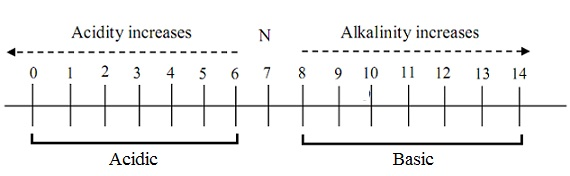#### pH notation and Buffer solution, Chemistry tutorial

Definition of pH:

pH can be simply be stated as the potential of hydrogen in a system. It exhibits the extent of acidity and basicity of a system under investigation.

Mathematically, pH is stated by sir Sorensor as the negative logarithm to base 10 of [H+].

That is, pH = - log [H+].

The only source of H+ is as an outcome of dissociation of water in which equivalent concentration of H+ and OH- are products.

H2O ↔ H+ + OH-

Water experiences self ionization termed as autoprotolysis in which, it acts as both acid and base.

H2O + H2O ↔ H3O+ + OH+

The autoprotolysis constant is equilibrium constant, KW, termed as the ionic product of water.

Kw = [H+][OH+]

This is for all time equivalents to 1.0 x 10-4 at 25oC

Taking log of both side, provides

log Kw = log [H+] + log [OH-]

- log Kw = - log [H+] + - log [OH-]

- log Kw = pH + pOH

pKw = pH + pOH

However at 25oC, pH = 7 and pOH= 7

Therefore, - log Kw = 14.00

That is, log10 = -14 or KW = 10-14

NB: The (-) negative sign is an indication that, concentration encountered is less than 1.0 M.

pKw = pH + pOH = 14.00

pH Scale:

A universal pH scale place the pH of most solution in the range 0.0 - 14.0. This must not provide an illusion that it is not possible to have negative pH (- pH). It simply means that the concentration hydrogen is more than 1M. This is attainable in concentrated solution of strong acid like HCl and H2SO4.Fig: pH Scale-Acidity and Alkalinity

NB-N= Neutrality

Measurement of pH:

pH is measured by a glass electrode. A glass electrode is the ion-selective electrode. This is extremely sensitive. The pH sensitive portion of the electrode is thin glass membrane which culminates in the slope of a bulb.

Most of the metal cation is positioned in the hydrated gels area of the metal membrane. These metals diffuse to the solution and are substituted by H+ in the solution in ion-exchange equilibrium. The more the H+ in solution, the more H+ ions are bound to the surface of glass.  The operation of glass electrode is symbolized as:

E = Constant + β (0.05915 log AH+ (outside)/AH+ (inside) at 25oC

Here 'β' is the electromotive efficiency = 1.00 or 0.9 8 and constant is termed as asymmetry potential.

pH at Elevated Temperature:

The major factor which finds out the real pH of a system is temperature. At high temperatures in a fluid system, the pH turns out to be high. Not only does pH influence the ionization of water in the body system, it as well changes the pH of a neutral solution to around 7.4, thus influencing the ionization constant of the acid and bases from which the buffer system is derived.

Buffer Solution:

A buffer solution can be stated as a solution which resists changes in pH whenever small amount of acid or base is added or whenever dilution takes place. Buffer solution consists of a mixture of a weak acid and its salt or a weak base and its salt.

Consider the acid HA undergoing dissociation under the equilibrium condition:

HA ↔ H+ + A-

Ka = [H-] [A-]/[HA]

log Ka = log [H-] [A-]/[HA] = log [H+] + log [A+]/HA

- log H+ = log Ka + log [A+]/[HA]

pH = PKa + log [salt]/[Acid]

The above is the Henderson- Hasselbalch equation

Buffer Capacity:

This is simply stated as the maximum amount of an acid or base which can be added to a buffer system devoid of causing a change or appreciable change in the pH of a system.

It will be noted that the buffering procedure for a mixture of a weak acid and its salt can be described as governed by log ratio of salt and acid.

pH = constant + log [A-]/[HA]

Whenever a solution is de-ionized, the ratio of pH remains constant. If a small quantity of strong acid is added, it will join with an equivalent amount of A- to convert it to HA.

The ratio of [A1] [HA] is small; therefore the change in pH is small, whereas if strong base is added it combines with [HA] to form an equivalent amount of A-. Again the change of pH is small.

Tutorsglobe: A way to secure high grade in your curriculum (Online Tutoring)